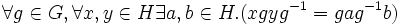# Conjugate-permutable subgroup

This article defines a term that has been used or referenced in a journal article or standard publication, but may not be generally accepted by the mathematical community as a standard term.[SHOW MORE]
This article defines a subgroup property: a property that can be evaluated to true/false given a group and a subgroup thereof, invariant under subgroup equivalence. View a complete list of subgroup properties[SHOW MORE]
This is a variation of permutability|Find other variations of permutability |

## History

### Origin

This term was introduced by: Tuval Foguel

Both the term and the concept of conjugate-permutable subgroups arose in the paper Conjugate-permutable subgroups by Tuval Foguel. Foguel introduced this notion by observing that the proof that permutable subgroups are subnormal actually generalizes to showing that conjugate-permutable subgroups are subnormal (in finite groups).

## Definition

### Symbol-free definition

A subgroup of a group is termed conjugate-permutable if it permutes with every conjugate of itself, or equivalently, if its product with every conjugate of it is a subgroup.

### Definition with symbols

A subgroup$H$ of a group$G$ is termed conjugate-permutable if$HH^g = H^gH$ for all$g$ in$G$, or equivalently, if$HH^g$ is a group for all$g$ in$G$.

(here$H^g = g^{-1}Hg$ is a conjugate subgroup to$H$ in$G$).

## Formalisms

BEWARE! This section of the article uses terminology local to the wiki, possibly without giving a full explanation of the terminology used (though efforts have been made to clarify terminology as much as possible within the particular context)

### First-order description

This subgroup property is a first-order subgroup property, viz., it has a first-order description in the theory of groups.
View a complete list of first-order subgroup properties

A subgroup$H$ is conjugate-permutable in a group$G$ if the following first-order sentence is satisfied:$\forall g \in G, \forall x,y \in H \exists a,b \in H . (xgyg^{-1} = gag^{-1}b)$

### Relation implication expression

This subgroup property is a relation implication-expressible subgroup property: it can be defined and viewed using a relation implication expression
View other relation implication-expressible subgroup properties

The property of being conjugate-permutable can be encoded as conjugate subgroups$\implies$ permuting subgroups. In other words$H$ is conjugate-permutable in$G$ iff any conjugate subgroup to$H$, permutes with$H$.

### In terms of the permutability operator

This property is obtained by applying the permutability operator to the property: conjugate subgroups
View other properties obtained by applying the permutability operator

The property of being conjugate-permutable is obtained by applying the permutability operator to the subgroup pair property of being conjugate.

## Metaproperties

Metaproperty name Satisfied? Proof Statement with symbols
transitive subgroup property No conjugate-permutability is not transitive It is possible to have groups$H \le K \le G$ such that$H$ is conjugate-permutable in$K$ and$K$ is conjugate-permutable in$G$, but$H$ is not conjugate-permutable in$G$.
intermediate subgroup condition Yes conjugate-permutability satisfies intermediate subgroup condition If$H$ is a conjugate-permutable subgroup of$G$, and$K$ is any intermediate subgroup of$G$, then$H$ is conjugate-permutable in$K$.
conjugate-join-closed subgroup property Yes conjugate-permutability is conjugate-join-closed If$H$ is a conjugate-permutable subgroup of$G$, then a join of conjugate subgroups to$H$ is again conjugate-permutable.
finite-join-closed subgroup property No conjugate-permutability is not finite-join-closed It is possible to have a group$G$ and conjugate-permutable subgroup$H,K$ of$G$ such that the join of subgroups$\langle H,K \rangle$ is not a conjugate-permutable subgroup.
finite-intersection-closed subgroup property No conjugate-permutability is not finite-intersection-closed It is possible to have a group$G$ and conjugate-permutable subgroup$H,K$ of$G$ such that the intersection of subgroups$H \cap K$ is not a conjugate-permutable subgroup.
directed union-closed subgroup property Yes conjugate-permutability is directed union-closed Suppose$(H_i)_{i \in I}$ is a directed set of subgroups of a group$G$, and every$H_i$ is conjugate-permutable in$G$. Then, the directed union of the$H_i$s is also conjugate-permutable in$G$.